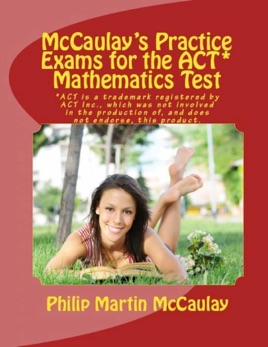• \$9.99

Publisher Description

McCaulay's Practice Exams for the ACT* Mathematics Test contains four complete 60-question, 60-minute sample tests for a total of 240 practice questions with answers and explanations designed to measure the mathematical skills students have typically acquired in courses taken by the end of 11th grade. The math content covered includes 23% Pre-Algebra, 17% Elementary Algebra, 15% Intermediate Algebra, 15% Coordinate Geometry, 23% Plane Geometry, and 7% Trigonometry. The questions on each exam are ordered by level of difficulty, from basic math problems to very challenging problems where one must plan an approach to solve them.